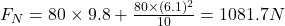## A circular-motion addict of mass 80 kg rides a Ferris wheel around in a vertical circle of radius 10 m at a constant speed of 6.1 m/s. (a) W

Question

A circular-motion addict of mass 80 kg rides a Ferris wheel around in a vertical circle of radius 10 m at a constant speed of 6.1 m/s. (a) What is the period of the motion? What is the magnitude of the normal force on the addict from the seat when both go through (b) the highest point of the circular path and (c) the lowest point?

in progress 0
6 months 2021-08-04T19:43:45+00:00 1 Answers 9 views 0

We are given that

Mass=80 kg

Speed,v=6.1 m/s

a.Time period,T=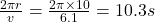b.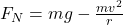Substitute the values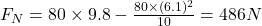c.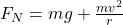Substitute the values